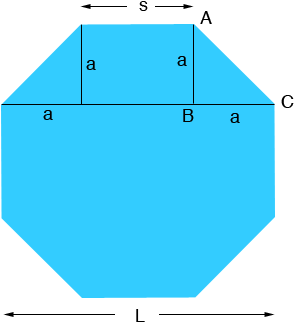Quandaries and Queries I understand there is a simple calculation to determine the sides of an octagon when you know the distance across the parallel flats. something like .447 . can you help? Private home owner with octagon skylights see www.domehome.ca for details. thanks Hi Royce, I copied one of the images of your skylight from your website.In the diagram below L is the distance between the sides and s is the length of a side.From the diagram L = s + 2 a Triangle ABC is a right triangle, and hence from Pythagoras Theorem a2 + a2 = s2 Thus a = s /√2 Hence L = s + 2 a = s + 2  s /√2 = s (1 + √2) = 2.4142 s or s = 0.4142 L Penny